﻿ Inverse Trigonometric Function: Arcsine

# Inverse Trigonometric Function: Arcsine

LESSON READ-THROUGH (Part 1 of 2)
by Dr. Carol JVF Burns (website creator)
Follow along with the highlighted text while you listen!
• PRACTICE (online exercises and printable worksheets)

Before studying this section, you are encouraged to read Trying to ‘Undo’ Trigonometric Functions.

For a function to have an inverse, each output must have exactly one corresponding input.
Thus, only one-to-one functions have inverses.

The sine function doesn't have a true inverse, because the sine function is not one-to-one.

So, to try and define an ‘inverse sine function’, we do the best we can, as discussed below.

 To try and define an ‘inverse sine function’, proceed as follows: Throw away most of the sine curve, leaving us with a piece that has three properties: the piece is one-to-one (and hence has an inverse) the piece covers all the outputs from the sine function (the interval $\,[-1,1]\,$) the piece is close to the origin The green piece shown at right satisfies all three of these properties. This green piece is the restriction of the sine curve to the interval $\,[-\frac{\pi}{2},\frac{\pi}{2}]\,.$ The function that the mathematical community calls ‘the inverse sine function’ is not actually the inverse of the sine function, because the sine function doesn't have a true inverse. Instead, the ‘inverse sine function’ is the inverse of this green piece of the sine curve. Several Cycles of the Graph of the Sine FunctionThe sine function isn't one-to-one; it doesn't pass a horizontal line test. So, it doesn't have a true inverse. To define an ‘inverse sine function’,we do the best we can. Throw away most of the curve—leave only the green part. This green part is one-to-one. This green part does have an inverse. The inverse of this green part is what the mathematical community calls ‘the inverse sine function’.

The arcsine function (precise definition below) is the best we can do in trying to get an inverse of the sine function.
The arcsine function is actually the inverse of the green piece shown above!

Here's a ‘function box’ view of what's going on:
 The sine function takes a real number as an input. It gives an output in the interval $\,[-1,1]\,.$ For example (as below), the output $\,0.5\,$ might come from the sine function.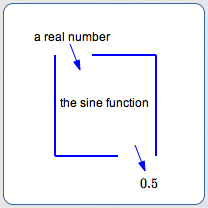When we try to use the sine function box ‘backwards’, we run into trouble. The output $\,0.5\,$ could have come from any of the inputs shown.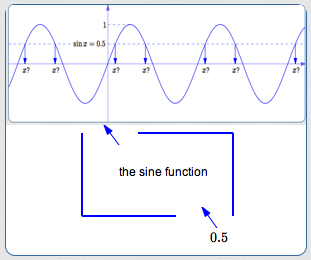However, when we use the green piece of the sine curve,the problem is solved! Now, there's only one input that works. (It's the value of the green $\,\color{green}{x}\,.$ ) Observe that $\,\color{green}{x}\,$ is in the interval $\,[-\frac{\pi}2,\frac{\pi}2]\,.$It's a bit of a misnomer, but the arcsine function (precise definition below) is often referred to as the ‘inverse sine function’.
A better name would be something like ‘the inverse of an appropriately-restricted sine function’.
(It's no surprise, however, that people don't say something that long and cumbersome.)

So, what exactly is $\,\arcsin 0.5\,$?

$\,\arcsin 0.5\,$ is the number in the interval $\,[-\frac{\pi}{2},\frac{\pi}{2}]\,$ whose sine is $\,0.5\,$

## What exactly IS $\,\arcsin x\,$?

More generally, let $\,x\,$ be any number in the interval $\,[-1,1]\,.$
Then:

$\,\arcsin x\,$ is the number in the interval $\,[-\frac{\pi}{2},\frac{\pi}{2}]\,$ whose sine is $\,x\,$

In my own mind (author Dr. Carol Burns speaking here), the words I say are:

$\,\arcsin x\,$ is the number between $\,-\frac{\pi}{2}\,$ and $\,\frac{\pi}{2}\,$ whose sine is $\,x\,$
I personally know the endpoints are included, so this doesn't confuse me.
However, the word ‘between’ is ambiguous—it can include the endpoints or not, depending on context.
It can be clarified by saying:
$\,\arcsin x\,$ is the number between $\,-\frac{\pi}{2}\,$ and $\,\frac{\pi}{2}\,$ (including the endpoints) whose sine is $\,x\,$
... but then it loses its simplicity. Ah—issues with language. Choose words that work for you!

## Precise Definition of the Arcsine Function

The precise definition of the arcsine function follows.
It can look a bit intimidating—the notes following the definition should help.

DEFINITION the arcsine function, denoted by  $\,\arcsin\,$  or  $\,\sin^{-1}\,$
Let $\,-1 \le x\le 1\,.$

Using the notation ‘$\,\arcsin\,$’ for the arcsine function: $$\cssId{s62}{y = \arcsin x}\ \ \ \ \ \cssId{s63}{\text{if and only if}}\ \ \ \ \cssId{s64}{\bigl(\ \sin y = x\ \ \text{AND}\ \ -\frac{\pi}{2} \le y\le \frac{\pi}{2}\ \bigr)}$$ Using the notation ‘$\,\sin^{-1}\,$’ for the arcsine function: $$\cssId{s66}{y = \sin^{-1} x}\ \ \ \ \ \cssId{s67}{\text{if and only if}}\ \ \ \ \cssId{s68}{\bigl(\ \sin y = x\ \ \text{AND}\ \ -\frac{\pi}{2} \le y\le \frac{\pi}{2}\ \bigr)}$$

## Notes on the Definition of the Arcsine Function:

• UNDERSTANDING THE DEFINITION:
The definition precisely answers the question: ‘What is the number $\,\arcsin x\,$?’
Here's how:
• Recall that ‘ if and only if ’ means the same thing as is equivalent to.
Equivalent sentences have identical truth values:
if one is true, so is the other;   if one is false, so is the other.
• Thus, if the sentence ‘$\,y = \arcsin x\,$’ is true,
then the compound sentence   ‘$\,\sin y = x\ \ \text{AND}\ \ -\frac{\pi}{2} \le y\le \frac{\pi}{2}\,$’   must also be true.
• If   ‘$\,y = \arcsin x\,$’   is true, then ‘$\,y\,$’ is just another name for ‘$\,\arcsin x\,$’.
• If   ‘$\,y = \arcsin x\,$’   is true, then two things must be true about $\,\arcsin x\,$ (i.e., $\,y\,$):
• $\,\sin \overbrace{y}^{\arcsin x} = x\,$:   its sine must be $\,x\,$
• $\,-\frac{\pi}{2} \le \overbrace{y}^{\arcsin x}\le \frac{\pi}{2}\,$:   it must be in the interval $\,[-\frac{\pi}{2},\frac{\pi}{2}]\,$
• Combining these ideas: $$\cssId{s88}{\overbrace{\strut y = \arcsin x}^{\text{How does a number get to be called \,\arcsin x\,?}}}\ \ \ \ \ \cssId{s89}{\overbrace{\strut \text{if and only if}}^{\text{The answer is:}}}\ \ \ \ \bigl(\ \cssId{s90}{\overbrace{\strut \sin y = x}^{\text{by having its sine equal to \,x\,}}}\ \ \cssId{s91}{\overbrace{\strut \text{AND}}^{\text{and}}}\ \ \cssId{s92}{\overbrace{\strut \ -\frac{\pi}{2} \le y\le \frac{\pi}{2}\ \ }^{\text{by being in the interval \strut \,[-\frac{\pi}{2},\frac{\pi}{2}]\,}}}\ \bigr)$$
• That is:
$\,\arcsin x\,$ is the number in the interval $\,[-\frac{\pi}{2},\frac{\pi}{2}]\,$ whose sine is $\,x\,$

• FORMAL NAME VERSUS FUNCTION NOTATION ABBREVIATIONS:
The formal name of the function being discussed in this section is ‘arcsine’.
It has an ‘e’ on the end, and is pronounced ARC-sine (with a long ‘i’ in ‘sine’).

When using function notation, ‘arcsine’ is abbreviated as ‘$\,\arcsin\,$’.
It has no ‘e’ on the end, but is pronounced the same as ‘arcsine’.
Thus, ‘$\,\arcsin x\,$’ is read aloud as ‘arcsine of $\,x\,$’.

An alternate notation for the arcsine function is ‘$\,\sin^{-1}\,$’.
This alternate notation is modeled on the standard notation for inverse functions:
if $\,f\,$ is one-to-one, then its inverse is called $\,f^{-1}\,.$

The function notation ‘$\,\sin^{-1} x\,$’ can be read aloud as ‘arcsine of $\,x\,$’ or ‘the inverse sine of $\,x\,$’.
DON'T read ‘$\,\sin^{-1} x\,$’ as ‘sine to the negative one of $\,x\,$’!
There is no reciprocal operation going on here—it's just standard notation for an inverse function.
• CONVENTION FOR MULTI-LETTER FUNCTION NAMES:
Since both ‘$\,\arcsin\,$’ and ‘$\,\sin^{-1}\,$’ are multi-letter function names, the standard convention applies:
the parentheses that typically hold the input can be removed, if there is no possible confusion about order of operations.

Thus, you usually see   ‘$\,\arcsin x\,$’   and   ‘$\,\sin^{-1} x\,$’   (no parentheses),
instead of the more cumbersome   ‘$\,\arcsin (x)\,$’   and   ‘$\,\sin^{-1} (x)\,$’   (with parentheses).
• FUNCTION NAME VERSUS OUTPUT FROM THE FUNCTION:
The function name is ‘$\,\arcsin\,$’.
The number ‘$\,\arcsin x\,$’ is the output from the function ‘$\,\arcsin\,$’ when the input is $\,x\,.$

Similarly, the function name is ‘$\,\sin^{-1}\,$’.
The number ‘$\,\sin^{-1} x\,$’ is the output from the function ‘$\,\sin^{-1}\,$’ when the input is $\,x\,.$
• PREFERRED NOTATION:
Since the sine function does not have a true inverse,
this author believes the notation ‘$\,\sin^{-1}\,$’ is misleading and lends itself to errors.
This author strongly prefers the notation ‘$\,\arcsin\,$’.
Inputs to trigonometric functions can be viewed as real numbers (radian measure) or degrees.
For example, $\,\sin \frac{\pi}{2} = 1\,$:   here, $\,\frac{\pi}{2}\,$ is radian measure.
Equivalently, $\,\sin 90^\circ = 1\,$:   here, $\,90^\circ\,$ is degree measure.

Here's what the definition of arcsine looks like, using degree measure instead of radian measure: $$\cssId{sb31}{y = \arcsin x}\ \ \ \ \ \cssId{sb32}{\text{if and only if}}\ \ \ \ \cssId{sb33}{\bigl(\ \sin y = x\ \ \text{AND}\ \ -90^\circ \le y\le 90^\circ\ \bigr)}$$
$\,\arcsin x\,$ is the angle in the interval $\,[-90^\circ,90^\circ]\,$ whose sine is $\,x\,$
• CALCULATOR SKILLS:
If a calculator is in degree mode, then $\,\arcsin x\,$ is reported in degrees.
If a calculator is in radian mode, then $\,\arcsin x\,$ is reported in radians.
• GRAPH OF THE ARCSINE FUNCTION:
For a one-to-one function $\,f\,,$ the graph of its inverse, $\,f^{-1}\,,$ is found by reflecting the graph of $\,f\,$ about the line $\,y = x\,.$
Below, this technique is used to construct the graph of the arcsine function:

 Here's the piece of the sine curve that is used to define the arcsine function:domain:   $\,[-\frac{\pi}{2},\frac{\pi}{2}]\,$ range:   $\,[-1,1]\,$ Here's the same curve, together with its reflection about the line $\,\color{red}{y = x}\,$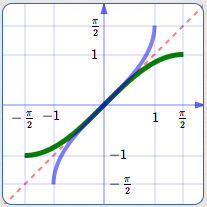The graph of the arcsine function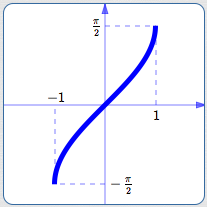domain:   $\,[-1,1]\,$ range:   $\,[-\frac{\pi}{2},\frac{\pi}{2}]\,$ Notice that the domain and range of a function and its inverse are switched! The domain of one is the range of the other. The range of one is the domain of the other.
• RELATIONSHIP BETWEEN THE SINE AND ARCSINE FUNCTIONS:
For a one-to-one function $\,f\,$ and its inverse $\,f^{-1}\,,$ there is a simple ‘undoing’ relationship between the two:
• $f^{-1}\bigl(f(x)\bigr) = x\,$ for all $\,x\,$ in the domain of $\,f\,$:
the function $\,f\,$ does something, and $\,f^{-1}\,$ undoes it
• $f\bigl(f^{-1}(x)\bigr) = x\,$ for all $\,x\,$ in the range of $\,f\,$:
the function $\,f^{-1}\,$ does something, and $\,f\,$ undoes it
Since the sine and arcsine functions are not true inverses of each other,
the relationship between them is a bit more complicated.

 Here's the direction where they do ‘undo’ each other nicely: start with a number, first apply the arcsine function, then apply the sine function, and end up right where you started. Here are the details: For all $\,x\in [-1,1]\,,$ $$\cssId{sb67}{\sin(\arcsin x) = x}$$ start with $\,\color{red}{x}\in [-1,1]\,$ the arcsine function takes $\,\color{red}{x}\,$ to $\,\color{green}{\arcsin x}\,$ in the interval $\,[-\frac{\pi}2,\frac{\pi}2]\,$ the sine function takes $\,\color{green}{\arcsin x}\,$ back to $\,\color{red}{x}\,$Here's the direction where they don't necessarily ‘undo’ each other nicely: start with a number, first apply the sine function, then apply the arcsine function. If the number you started with is outside the interval $\,[-\frac{\pi}2,\frac{\pi}2]\,,$ then you don't end up where you started! Here are the details: For all $\,x\in [-\frac{\pi}2,\frac{\pi}2]\,,$ $$\cssId{sb77}{\arcsin(\sin x) = x}$$ (See the top graph at right.) For all $\,x\not\in [-\frac{\pi}2,\frac{\pi}2]\,,$ $$\cssId{sb80}{\arcsin(\sin x) \ne x}$$ (See the bottom graph at right.)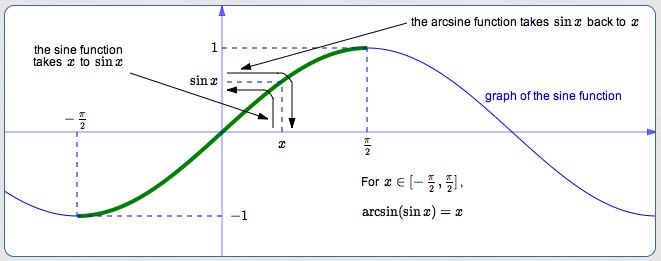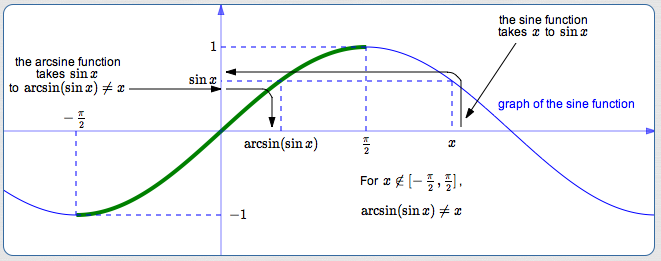## Example: Find the Exact Value of $\,\arcsin(-0.5)\,$ (use both the unit circle and a special triangle)

 Using the degree definition: $\,\arcsin (-\frac 12)\,$ is the angle between $\,-90^\circ\,$ and $\,90^\circ\,$ whose sine is $\,-\frac 12\,.$ Recall: sine is the $y$-value of points on the unit circle. Draw a unit circle. Mark $\,-\frac 12\,$ on the $y$-axis. Mark the unique angle between $\,-90^\circ\,$ and $\,90^\circ\,$ that has this sine value. This (negative) angle is $\,\arcsin(-\frac 12)\,.$ Does any special triangle tell us an acute angle whose sine is $\,\frac12\,$? Yes! The sine of $\,30^\circ\,$ is $\,\frac 12\,.$ Thus, $\,\arcsin(-\frac 12) = -30^\circ\,.$ Using radian measure, $\,\arcsin(-\frac 12) = -\frac{\pi}6\,.$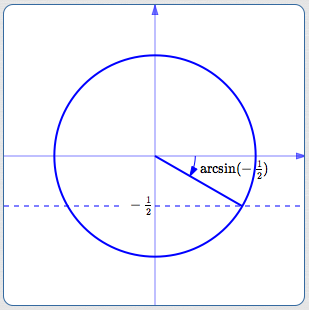Master the ideas from this section